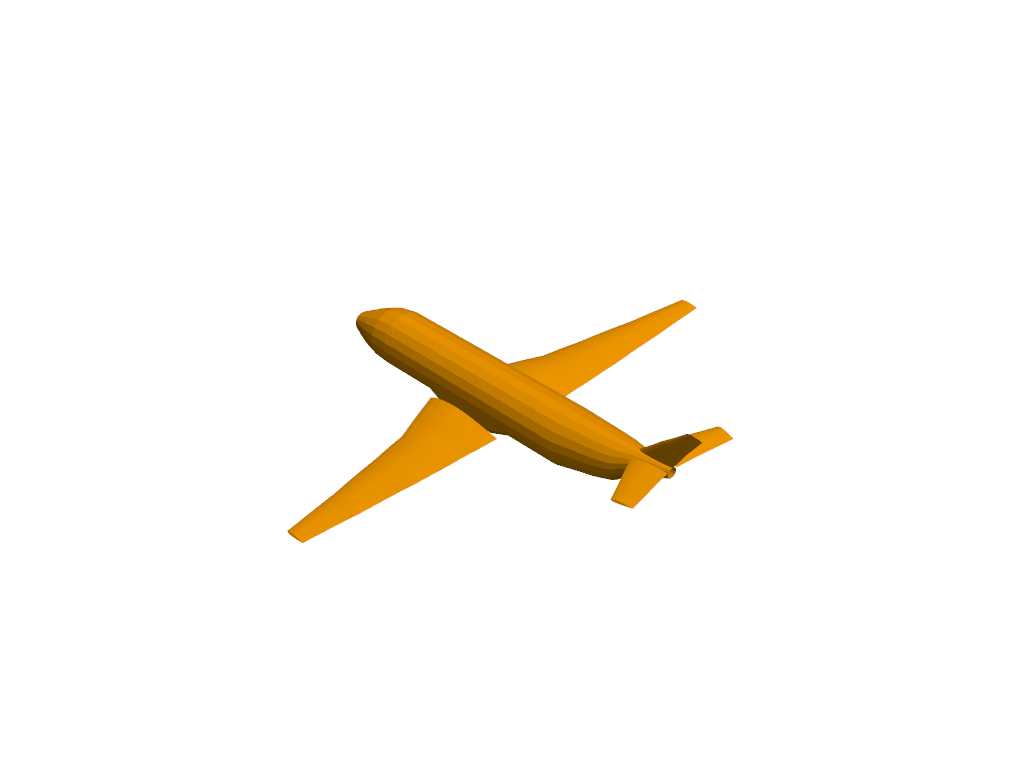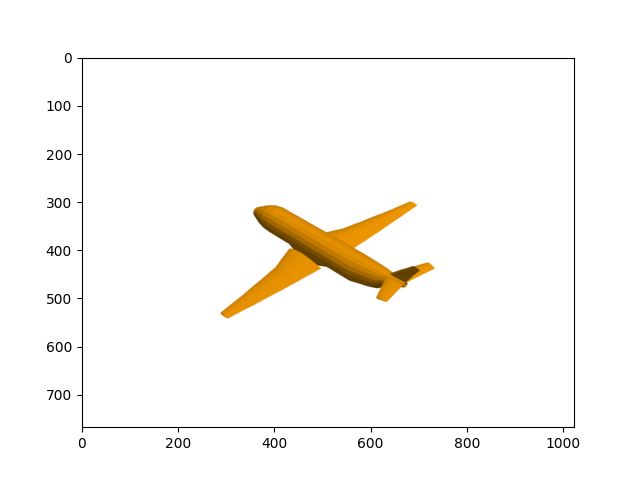# Saving Screenshots¶

```# sphinx_gallery_thumbnail_number = 2
import pyvista as pv
from pyvista import examples
import numpy as np
import matplotlib.pyplot as plt

# Get a sample file
filename = examples.planefile
```

You can also take a screenshot without creating an interactive plot window using the `pyvista.Plotter`:

```plotter = pv.Plotter(off_screen=True)
plotter.show(screenshot='airplane.png')
```Out:

```[(3140.593704331348, 2919.620300400684, 2375.7925778512454), (896.9955291748047, 676.0221252441406, 132.19440269470215), (0.0, 0.0, 1.0)]
```

The `img` array can be used to plot the screenshot in `matplotlib`:

```plt.imshow(plotter.image)
plt.show()
```Out:

```/home/travis/build/pyvista/pyvista/examples/02-plot/screenshot.py:28: UserWarning: Matplotlib is currently using agg, which is a non-GUI backend, so cannot show the figure.
plt.show()
```

Total running time of the script: ( 0 minutes 1.337 seconds)

Gallery generated by Sphinx-Gallery